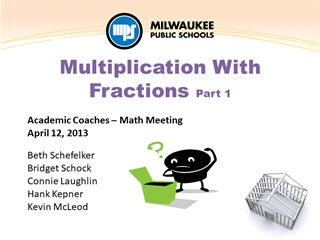# Fractions - Multiplication - Academic Coaches – Math Meeting April 12, 2013 Beth Schefelker

0

#### Presentation Transcript

• 1. Academic Coaches – Math Meeting April 12, 2013 Beth Schefelker Bridget Schock Connie Laughlin Hank Kepner Kevin McLeod Multiplication With Fractions Part 1
• 2.Professional Practice from March: Engage in a coaching conversation with your teachers around these ideas. Operating with Fractions Progression Multiplication Situations Turn and Talk Share an “a-ha” moment from the conversation. Either your “a-ha” or your teacher’s “a-ha”. Sharing Coaching Experiences
• 3.Does Order Matter? What does it mean to multiply a fraction by a whole number? What does it mean to multiply a whole number by a fraction?
• 4.Learning Intentions & Success Criteria We are learning to …. Understand multiplication with fractions using meaningful visual models and real-world contexts. We will be successful when we can …. Recognize, represent, and contextualize ‘groups of’ and ‘parts of’ problems involving multiplication with fractions.
• 5.Ribbon and Bows Connie is making bows for her grandchildren. Each bow takes ¾ yard of ribbon. She needs 7 bows. How many yards of ribbon does she need? Make a visual representation Write an equation that represents the problem Solve the problem
• 6.One Meaning of Multiplication with Fractions: ‘Groups of’ Problems 6 x ⅔ 6 groups of ⅔ Tell a story  Draw a representation of your story 4.NF.4 Apply and extend previous understandings of multiplication to multiply a fraction by a whole number. (c) Solve word problems involving multiplication of a fraction by a whole number, e.g., by using visual fraction models and equations to represent the problem.
• 7.House For Sale! Elena and Roberto are deciding which house to buy. The first house has a lot that is 6 acres. The second house’s lot is as large. What is the size of the second lot?  Make a visual representation Write an equation that represents the problem Solve the problem
• 8. x 4  part of 4 Tell a story  Draw a representation of your story Second Meaning of Multiplication of Fractions:‘Parts of’ Problems 5.NF.4 Apply and extend previous understandings of multiplication to multiply a fraction or whole number by a fraction.
• 9.Does Order Matter? What does it mean to multiply a fraction by a whole number? What does it mean to multiply a whole number by a fraction?
• 10.‘Groups of’ vs. ‘Parts of’ Work in your table groups: Sort the cards as a ‘Groups of’ problem or a ‘Parts of’ problem. Use a representation to help you sort the cards. As you worked through the problems, how did your thinking evolve?
• 11.Learning Intentions & Success Criteria We are learning to …. Understand multiplication with fractions using meaningful visual models and real-world contexts. We will be successful when we can …. Represent, contextualize, and justify ‘groups of’ and ‘parts of’ problems involving multiplication with fractions.
• 12.Reflection Consider these two Standards for Mathematical Practice: MP2. Reason abstractly and quantitatively. MP6. Attend to precision. Select one, describe how this standard was evident when multiplying fractions. Use specific examples to explain your reasoning.
• 13.Professional Practice Use a variety of resources to find contextual situations involving multiplication with fractions. Bring a sample of a ‘groups of’ and ‘parts of’ problem to the April 26th ACM meeting to share.